Mechanics

# ABS brakes

ABS brakes are more efficient than conventional brakes, as they do not cause the wheels to lock, but work by reducing rotation, preventing slippage.

The ABS ( Antilock Brake System ) braking system is more efficient than conventional drum or disc brakes, as they do not lock the wheels, which prevents the tires from sliding on the asphalt.

The braking space, the space needed for the vehicle to stop, is significantly reduced with the use of ABS brakes, which makes this type of equipment safer. With this in mind, in 2014, the National Traffic Council (Contran) established that all cars manufactured in Brazil must have airbags and ABS brakes.

braking space

The braking space is the distance required for a vehicle to stop after applying the brakes. Locking the wheels makes the tires slide on the asphalt, leaving the braking space higher. ABS brakes work with the reduction of wheel rotation and prevent them from locking, thus, sliding on the ground is prevented and braking space is reduced.

The determination of the braking space is done through the Torricelli equation :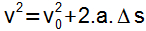The terms of this equation have the following definition:

v = Final speed of the mobile (m/s);

0 = Initial velocity of the mobile (m/s);

a = Acceleration (m/s 2 );

Δs = Distance traveled (m).

The final speed of the car after braking is zero, the acceleration must be negative, as the vehicle undergoes a deceleration, and the space covered is precisely the so-called braking space. In this way, one has to: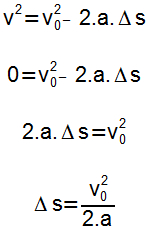From the definition of Newton’s second law and knowing the idea of ​​friction force , we can determine the acceleration as follows: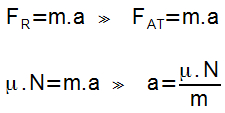Knowing that, in this case, the normal force (N) can be replaced by the weight force , one can substitute the previous definition of acceleration in the equation that determines the braking space. So we will have: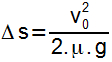The quantity represented by μ corresponds to the coefficient of friction , a dimensionless quantity that characterizes a surface, indicating whether it offers much or little friction. If an object is at rest on a given surface, the coefficient of friction is called static μ E ) . If the object is moving, the friction forces will have a lower value and the coefficient will be defined as the coefficient of kinetic friction ( μ C ) .

The values ​​of the coefficients of static friction are always greater than the values ​​of the coefficient of kinetic friction. It can be seen that the effort required to put an object in motion is greater than that made to keep it in motion, which highlights this difference. In this way, we can write that: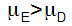If there is slippage between the tires and the ground, the friction will be classified as dynamic, as a single point of the tire is dragged. ABS brakes do not allow the wheels to lock, thus, at each moment, a different point of the tire is on the ground, which determines the existence of static friction. Note that the braking space is inversely proportional to the friction coefficient, therefore, the static friction, being greater, determines a smaller braking space.

The operation of ABS brakes provides the prevalence of static friction, which reduces the braking space, brings safety to vehicle passengers and prevents traffic accidents.